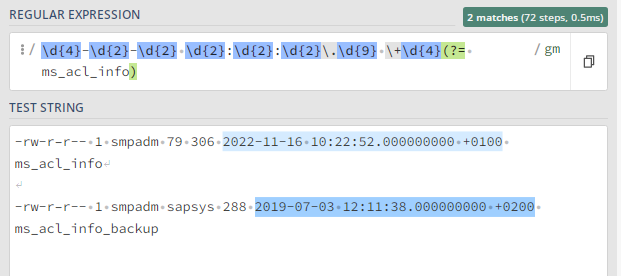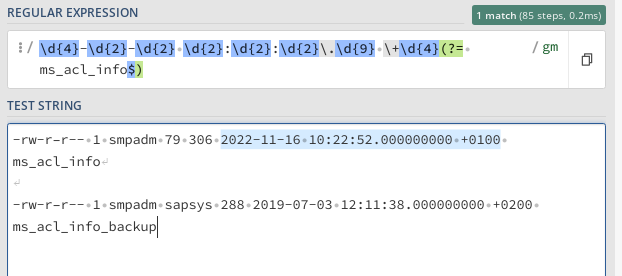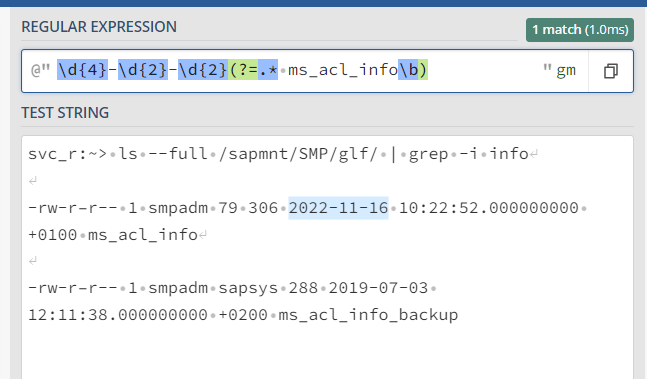# Regex to capture only date from the string

The date format has been changed recently for the text captured… since then the below regex is not working

Please provide the regex format to capture only the text in the “ms_acl_info” ROW

Captured Text:
svc_r:~> ls --full /sapmnt/SMP/glf/ | grep -i info

-rw-r–r-- 1 smpadm 79 306 2022-11-16 10:22:52.000000000 +0100 ms_acl_info

-rw-r–r-- 1 smpadm sapsys 288 2019-07-03 12:11:38.000000000 +0200 ms_acl_info_backup

Current reqx : (?[A-Z][a-z]{2} {1,2}\d{1,2} {1,2}(\d{0,4} )?)(\d{2}:\d{2} )?(?ms_acl_info)\r?\$

Hey,

You can use the following RegEx expression to fetch the desired data to an extent:

`\d{4}-\d{2}-\d{2}\s\d{2}:\d{2}:\d{2}\.\d{9}\s\+\d{4}(?=\s+ms_acl_info\$)`

This will check for the line which ends with ms_acl_info & gives the output as 2022-11-16 10:22:52.000000000 +0100, from which you can easily extract the data either by using string manipulation or another simple RegEx.

Hope this helps,
Best Regards.

Hi @Sathish_Kumar_S ,
try below regex pattern
`\d{4}-\d{2}-\d{2} \d{2}:\d{2}:\d{2}\.\d{9} \+\d{4}(?= ms_acl_info)`Regards,
Arivu

Hi @Sathish_Kumar_S ,
try below regex pattern
`\d{4}-\d{2}-\d{2} \d{2}:\d{2}:\d{2}\.\d{9} \+\d{4}(?= ms_acl_info)`If you want only `ms_acl_info` use below regex
`\d{4}-\d{2}-\d{2} \d{2}:\d{2}:\d{2}\.\d{9} \+\d{4}(?= ms_acl_info\$)`Regards,
Arivu

1 Like

how to capture only the date ( exclude the time)

`\d{4}-\d{2}-\d{2}(?=.* ms_acl_info)`

`\d{4}-\d{2}-\d{2}(?=.* ms_acl_info\$)`

Regards,
Arivu

1 Like

To capture date only excluding the time, try this:
\d{4}-\d{2}-\d{2}\s

1 Like

now it is highlighting only date but is highlinghting the date in both lines

We need the date only from the line ms_acl_info.

Also it is highlighting only if i remove \$ in the end

Could you try using the below Regex Expression :

``````\d{4}-\d{2}-\d{2}(?=.* ms_acl_info\b)
``````1 Like

Thankyou @supermanPunch

it works … how do i assign the captured regex value to string variable?

Datecaptured variable is string and here is the expression :

System.Text.RegularExpressions.Regex.Match(MatchItem.ToString,“+\s\d+”).Tostring

when we assign the “Matchitem” to string… it is giving empty value

1. a-zA-Z ↩︎

Why do you use another Regex Expression again ?

Try just using `MatchItem.Value` and check if you are able to get the result.

This topic was automatically closed 3 days after the last reply. New replies are no longer allowed.